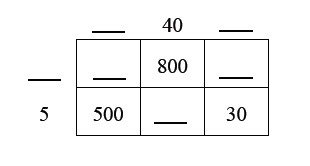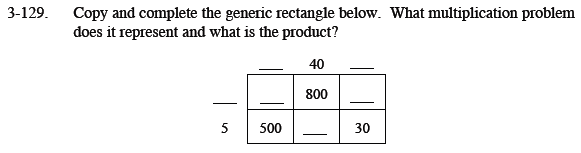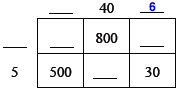### Home > MC1 > Chapter 3 > Lesson 3.4.4 > Problem3-129

3-129.

. Copy and complete the generic rectangle below. What multiplication problem does it represent and what is the product? Homework Help ✎If you are having trouble getting started with the generic rectangle, refer to problem 3-63.
This problem is a bit different, since some of the numbers will be above 100.

For generic rectangles, remember that the product it represents is simply the sum of the areas within the rectangle.

To get started, here is one of the missing numbers. Can you see why it is correct?